# Maharashtra Board Class 9 Maths Solutions Chapter 4 Ratio and Proportion Practice Set 4.3

## Maharashtra Board Class 9 Maths Solutions Chapter 4 Ratio and Proportion Practice Set 4.3

Question 1.
If $$\frac { a }{ b }$$ = $$\frac { 7 }{ 3 }$$, then find the aIues of the following ratios.Solution: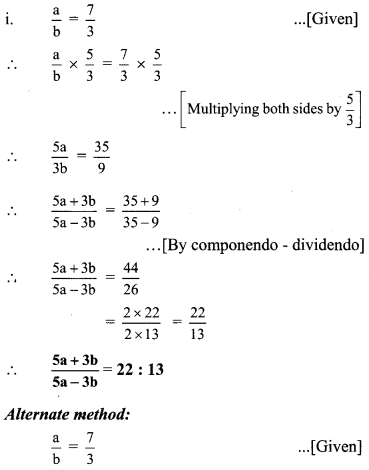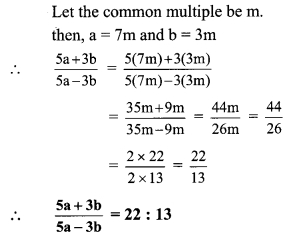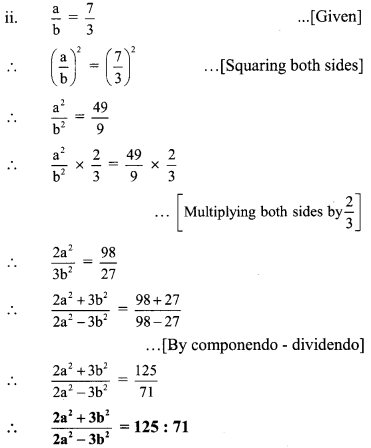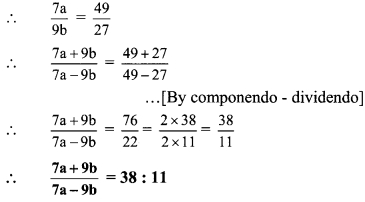Question 2.
If $$\frac{15 a^{2}+4 b^{2}}{15 a^{2}-4 b^{2}}=\frac{47}{7}$$, then find the value of the following ratios.Solution: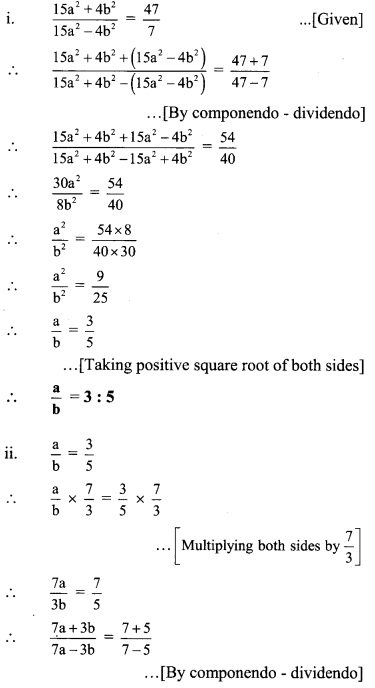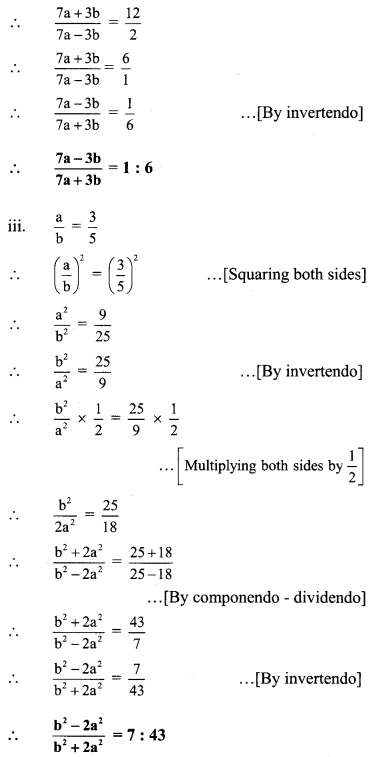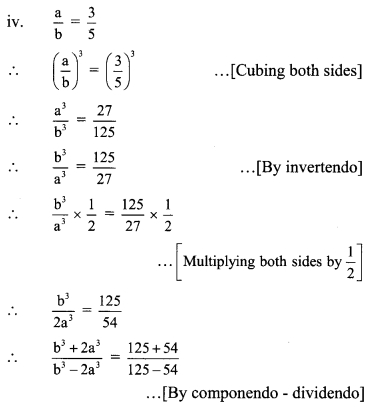Question 3.
If $$\frac{3 a+7 b}{3 a-7 b}=\frac{4}{3}$$then find the value of the ratio $$\frac{3 a^{2}-7 b^{2}}{3 a^{2}+7 b^{2}}$$.
Solution: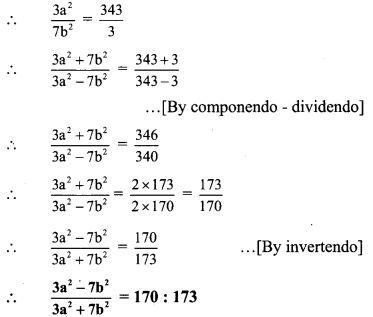Question 4.
Solve the following equations.Solution: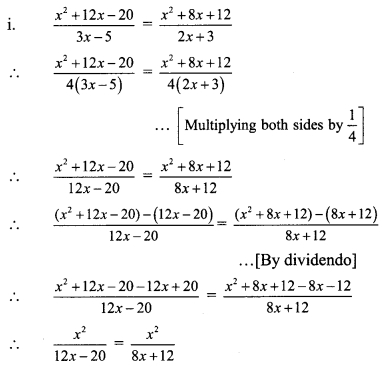This equation is true for x = 0
∴ x = 0 is one of the solutions.
If x ≠ 0, then x2 ≠ 0
∴ $$\frac { 1 }{ 12x – 20 }$$ = $$\frac { 1 }{ 8x + 12 }$$ … [Dividing both sides by x2]
∴ 8x + 12 = 12x – 20
∴ 12 + 20 = 12x – 8x
∴ 32 = 4x
∴ x = 8
∴ x = 0 or x = 8 are the solutions of the given equation.∴ 21(x – 5) = 4(2x + 3)
∴ 21x – 105 = 8x + 12
∴ 21x – 8x = 12 + 105
∴ 13x = 117
∴ x = 9
∴ x = 9 ¡s the solution of the given equation.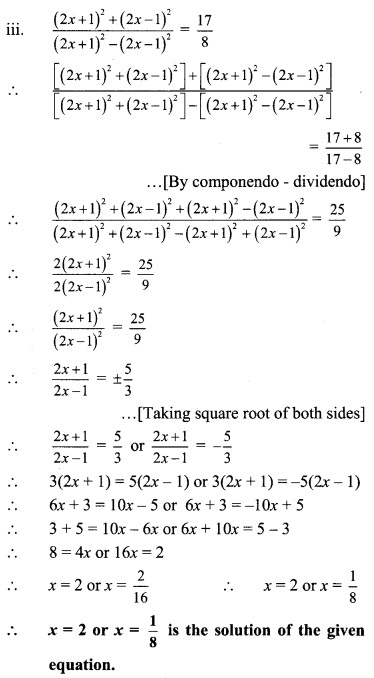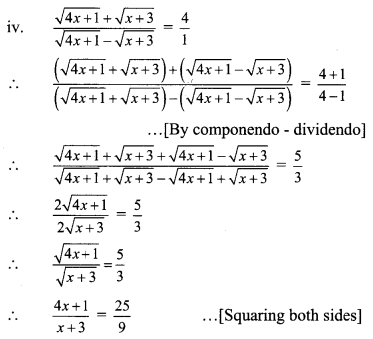∴ 9(4x + 1) = 25(x + 3)
∴36x + 925x + 75
∴ 36x – 25 = 75 – 9
∴11x = 66
∴ x = 6
∴ x = 6 is the solution of the given equation.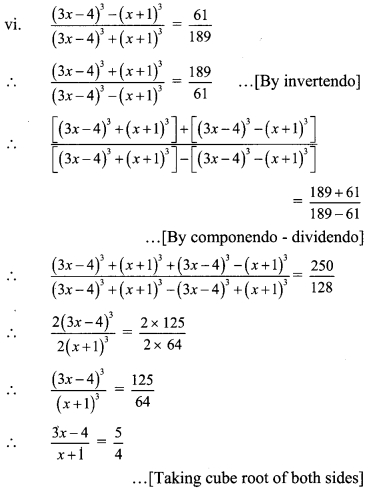∴ 4(3x – 4) = 5(x + 1)
∴ 12x – 16 = 5x + 5
∴ 12x – 5x = 5 + 16
∴ 7x = 21
∴ x = 3
∴ x = 3 ¡s the solution of the given equation.